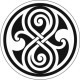# LOG#146. Path integral (I).My next thematic thread will cover the Feynman path integral approach to Quantum Mechanics!

The standard formulation of Quantum Mechanics is well known. It was built and created by Schrödinger, Heisenberg and Dirac, plus many others, between 1925-1931. Later, it was shown to be equivalent and analogue to the common Classical Mechanics (Dirac, Von Neumann,…). In 1933, Dirac made the remarkable observation that the action plays a central role in classical mechanics but that it seemed to have no important role in Quantum Mechanics so far. However, he speculated on how this situation could be solved, and he suggested that the propagator (Green’s function), essentially the matrix elements of the scattering matrix (S-matrix) in momentum space, in Quantum Mechanics should be proportional to the phase factor

(1)and where S is the classical action evaluated along the classical path.

In 1948, Feynman developed Dirac’s idea, and he succeeded in his new approach: he was able to provide a third formulation of Quantum Mechanics, based on the fact that the propagator can be written as a sum over ALL (not a single one!) the possible paths (not only the classical path!) between the initial and final points. Each path contributes with a weight and the probability, the squared amplitude, reads:

(2)There is a difference between Dirac idea and the final output by Feynman. Dirac considered only the classical path, while Feynman showed that ALL paths contribute. In some sense, the quantum particle “smells” and “takes” all paths and interferes with itself, so the amplitudes for every path add according to the usual quantum mechanical rule for combining amplitudes, i.e., the rule of superposition and then it gets squared.

It should be also remembered that  the developments about the Brownian motion in the period 1900-1930 were very important in that period. Its rigorous mathematical formulation and foundation (Wiener et alii, as “Wiener processes”) was very relevant here. Wiener himself showed that the Schrödinger differential equation and its solution can be understood in terms of the average of some stochastic equation. It is called Wiener process Brownian motion. Nowadays, Wiener idea is the basis for the rigorous path integral formulation of Quantum Mechanics with the aid of the Feynman-Kac calculus and its formulae.

Wiener processes are important in different areas:

1st. Pure mathematical sciences.

2nd. Brownian motion theory: diffusion via the Fokker-Planck and Langevin equations.

3rd. Finance. The “Black-Scholes” equation (option pricing models) and in general any theory of complex systems with infinite or nearly infinite degrees of freedom can be modeled by path integrals.

Let me add some extra motivations. Path integrals give us no new results in the quantum mechanics of a single particle. So, why is it so useful? After all, not every calculation in Quantum Mechanics done by path integrals can be done without it. The key point is that of simplicity and covariance. Path integrals provide a powerful and physically appealing, intuitive and formidable way of viewing Quantum Mechanics. The classical limit of Quantum Mechanics can be understood in a particularly simple and clean way from path integrals. Even the classical principle of minimal action can be understood (and “explained”!) from this new approach!

Path integrals turn out to be considerably more useful in some complex problems and situations, such as gauge field theory and particle physics  or condensed matter physics. In Quantum Field Theory (QFT), both relativistic and nonrelativistic, path integrals (or functional integrals) play a very important role. The reasons are easy to follow up:

1st. Path integral provide a relatively easy road to quantization and to write Green’s functions. Green functions or propagators are related to amplitudes for physical processes such as scattering or decays.

2nd. The path integral formulation of gauge theories (non-abelian and abelian) is very elegant: it allows gauge fixing and the ghost fields arise naturally.

3rd. There are a whole set of nonperturbative phenomena such as solitons and instantons that are most easily studied with the path integral or functional methods.

4th. There is a close link between statistical mechanics and quantum mechanics with the path integral approach. The relationship between these two fields is seen with a clean correspondence between path integrals and partition functions.

In this thread I am going to discuss the following topics:

i) Functionals. Gaussian functionals and integrals.

ii) Path integrals in Quantum Mechanics. Some examples as the free particle or the harmonic oscillator.

iii) How to “derive” classical mechanics from quantum mechanics, i.e., how to understand the classical minimal action from the path integral formalism.

iv) Statistical mechanics and path integrals: the nexus between path integrals and the partition function of classical statitiscal mechanics.

v) Perturbation theory in quantum mechanics.

vi) Green functions or propagators in Quantum Mechanics. Propagators as vacuum expectation values of time-ordered operator products. Field theory via path integrals.

a) Find out the original papers by Dirac and Feynman.

b) Search for the link between Wiener processes and the Brownian motion.

c) Find out and write the Fokker-Planck equation.

d) Search for the Langevin equation.

e) Search for the “Black-Scholes” equation and its application in mathematical finances.

f) Find the application of path integrals in some complex problems like the Aharanov-Bohm effect, quantum statistics of anyons and the link between charge quantization and magnetic monopoles.

See you in my next TSOR post!

#### LOG#146. Path integral (I). — 2 Comments

1.Peter on said:

I enjoyed this page, and especially the two diagrams – did you come mup with them?

atb
peter

•amarashiki on said:

The diagrams are not mine, I found them using google images, and one of them is by Sean Carroll, the famous cosmologist…

This site uses Akismet to reduce spam. Learn how your comment data is processed.